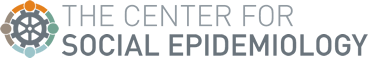The reference for the following graphic is: Landsbergis PA, Schnall PL, Schwartz JE, Pickering TG, Warren K. The association of ambulatory blood pressure with alternative forms of job strain. Scand J Work Env Health; 20:349-63,1994.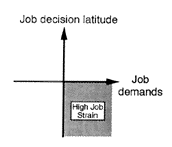Quadrant formed by dichotomizing latitude and demands scores at the medians of the current sample (latitude <74 and demands >32).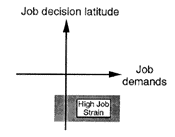Quadrant term using national means

Area formed by using national (U.S. QES) means for latitude and demands (latitude <72.6 and demands>30.6 for males: latitude <65.7 and demands > 31.3 for females).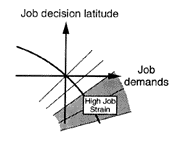Quotient term

Sample demands divided by latitude. This continuous form of the variable can be dichotomized at an arbitrary cutpoint.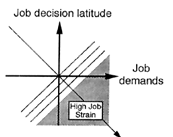Linear term

Linear function created by y=(b) demands-(c) latitude. (If both variables are on the same scale, y=(0.5) demands – (0.5) latitude.) This continuous form of the variable can be dichotomized at an arbitrary cutpoint.

Note: The cutpoints for job decision latitude listed above reflect corrections made for the U.S. QES means for the job decision latitude scale score in December 2000. In addition, the cutpoints for job decision latitude listed above are based on the formulas in the Job Content Questionnaire manual in which the job decision latitude scale score is multiplied by 2. Papers published from the Cornell Blood Pressure Study used a different version of the formula in which the job latitude scale score was not multiplied by 2. Thus, for anyone wishing to compare their findings to the Cornell study findings, do not multiply the job decision latitude by 2and use the following cutpoints:
1) for the job strain quadrant term based on Cornell sample medians, use latitude <37 and demands >32.
2) for the job strain quadrant term using national means, use latitude <36.3 and demands >30.6 for males; latitude <32.9 and demands >31.3 for females.